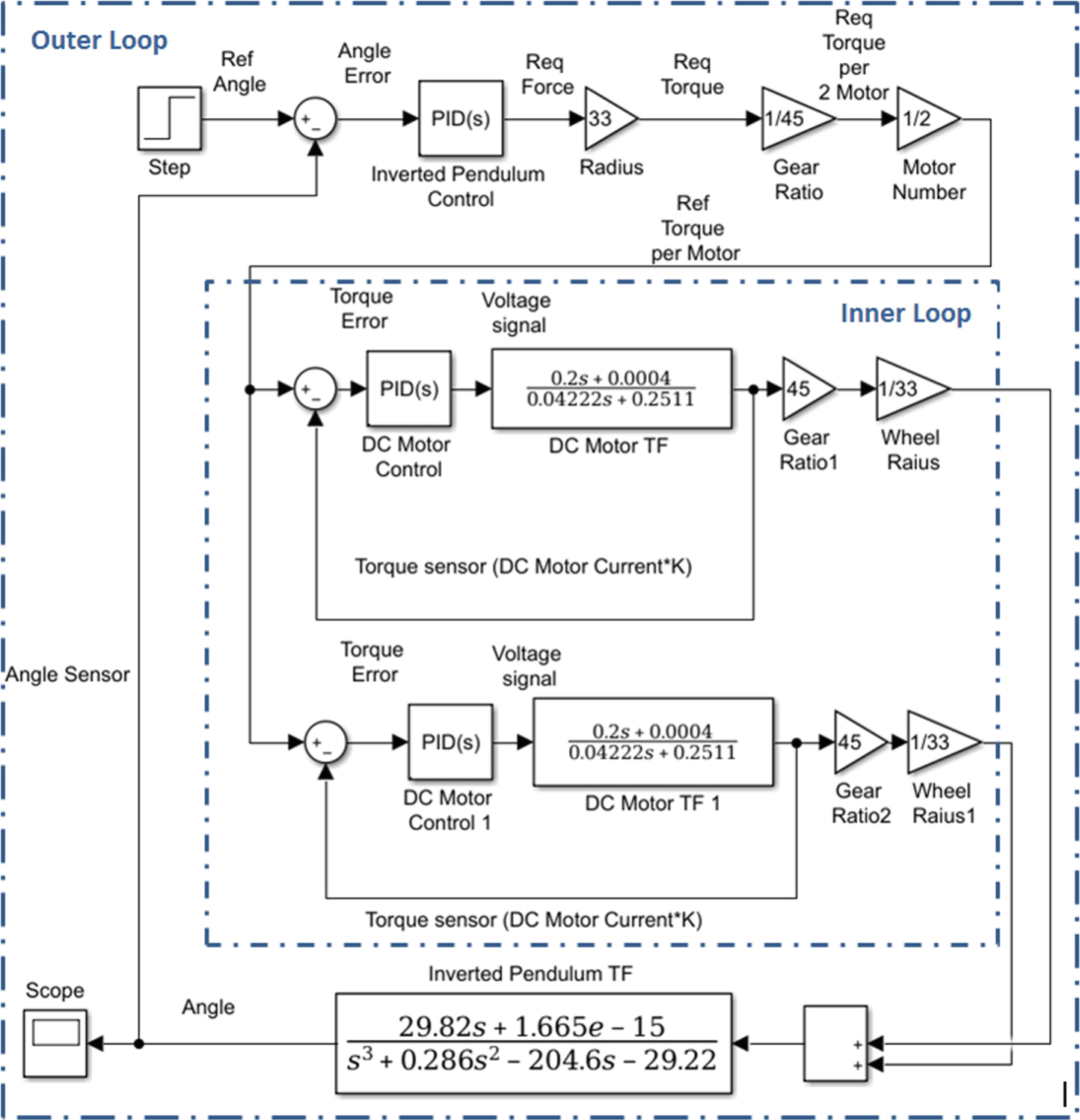Conference Paper

# Self-balancing Robot Modeling and Control Using Two Degree of Freedom PID Controller

By
Azar A.T.
Ammar H.H.
Barakat M.H.
Saleh M.A.
Abdelwahed M.A.

This paper represents the control of a two-wheel self-balancing robot based on the theory of controlling the inverted pendulum. This paper dividing the system modeling into two main parts. The first part is the dc motor and the second part are the whole mechanical design and its characteristics as a function in the motor speed and the torque depending on the system, creating two control closed loops inner and outer. The study uses conventional proportional–integral–derivative (PID) and two degree of freedom PID controllers to obtain a robust controller for the system. The inner loop controls the motor speed use the motor speed feedback signal from the encoder. The outer loop keeps the robot always in the accepted vertical angle boundary, using a six-degree of freedom gyroscope and accelerometer as a feedback signal. A state space model is obtained considering some assumptions and simplifications. The results are verified through simulations and experiments. Numerical simulation results indicate that the 2-DOF PID controller is superior to the traditional PID controller. © Springer Nature Switzerland AG 2019.